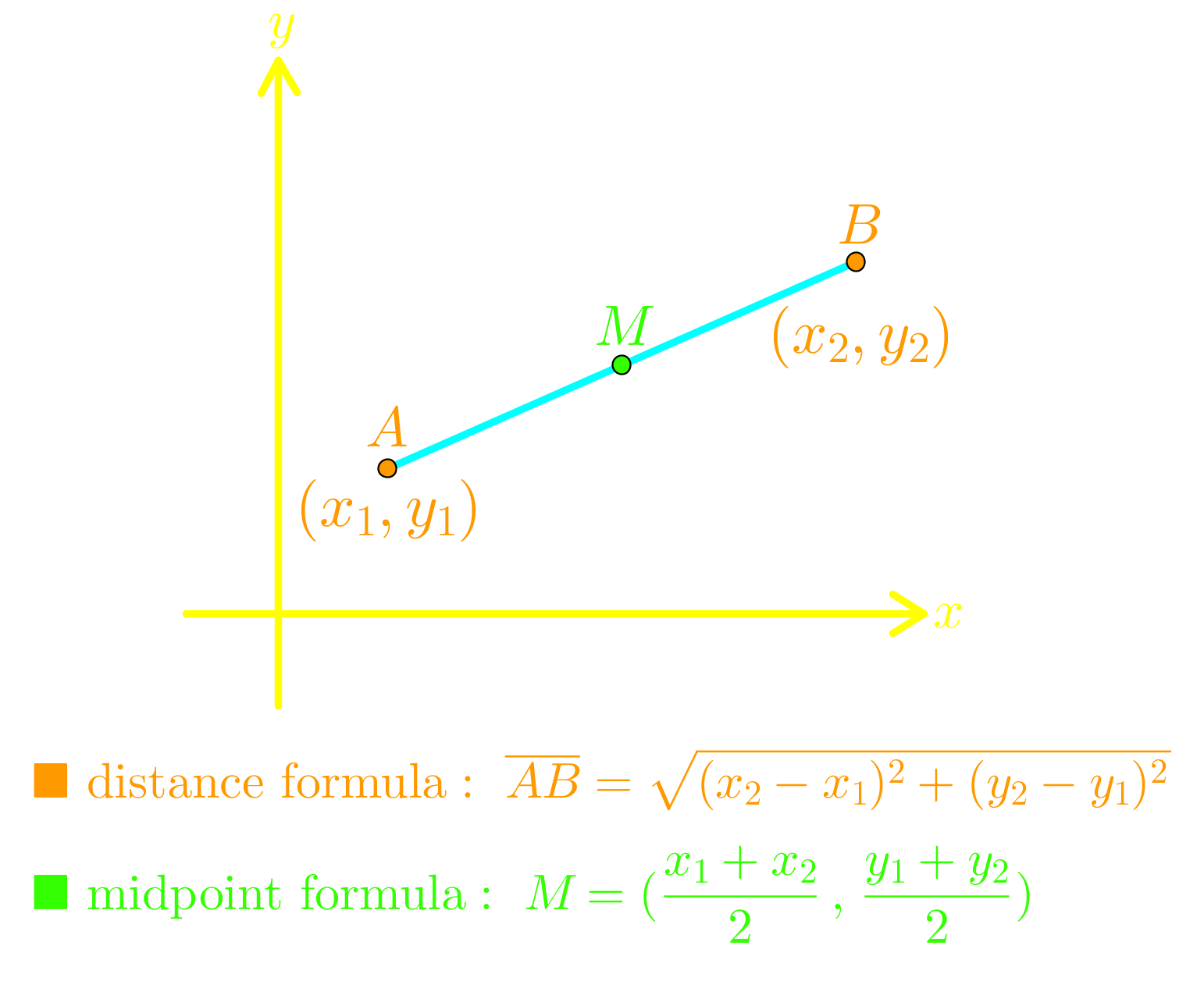# Conics - Circle

0/8
##### Examples
###### Lessons
1. graphing a circle
Sketch each circle and state the:
i) center
1. ${\left( {\frac{{x - 5}}{3}} \right)^2} + {\left( {\frac{{y + 4}}{3}} \right)^2} = 1$
2. ${x^2} + {y^2} = 25$
2. converting a circle equation to conics form by "completing the square"
$4{x^2} + 4{y^2} + 24x - 8y + 15 = 0$
1. Convert the equation to conics form.
2. Sketch the graph and state the:
i) center
3. finding the equation of a circle given its properties
Find the equation of a circle with:
1. center $\left( { - 3,\;5} \right)$, radius = 7
2. center $\left( {2,\;0} \right)$, passing through the point $\left( { - 1,\;4} \right)$
3. diameter with endpoints $\left( { - 9,\;4} \right)$ and $\left( {15,\; - 6} \right)$
4. center $\left( { - 2,\; - 1} \right)$, tangent to the line $3x + 4y = 15$
###### Free to Join!
StudyPug is a learning help platform covering math and science from grade 4 all the way to second year university. Our video tutorials, unlimited practice problems, and step-by-step explanations provide you or your child with all the help you need to master concepts. On top of that, it's fun - with achievements, customizable avatars, and awards to keep you motivated.
• #### Easily See Your ProgressWe track the progress you've made on a topic so you know what you've done. From the course view you can easily see what topics have what and the progress you've made on them. Fill the rings to completely master that section or mouse over the icon to see more details.
• #### Make Use of Our Learning Aids###### Practice Accuracy

See how well your practice sessions are going over time.

Stay on track with our daily recommendations.

• #### Earn Achievements as You LearnMake the most of your time as you use StudyPug to help you achieve your goals. Earn fun little badges the more you watch, practice, and use our service.
• #### Create and Customize Your AvatarPlay with our fun little avatar builder to create and customize your own avatar on StudyPug. Choose your face, eye colour, hair colour and style, and background. Unlock more options the more you use StudyPug.
###### Topic NotesThe conics form of a circle with center $\left( {h,\;k} \right)$ and radius $r$ is:
${\left( {\frac{{x - h}}{r}} \right)^2} + {\left( {\frac{{y - k}}{r}} \right)^2} = 1$